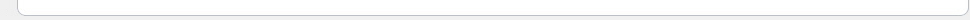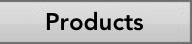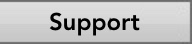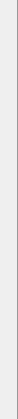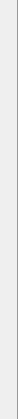# Statistics Pro - The Calculator of Choice for Statistics, Business Statistics, and Discrete Mathematics/Structures!

Statistics is a great class, but the nature of the calculations in homework, quizes and tests can be tedious and time-consuming.  You can fully understand the formula you're working with and the calculation you're doing, but one slight miscalculation will affect the entire formula and produce a wrong answer!

Modern calculators still require tons of button-mashing, and scientific calculators are expensive and may require you to study a 100+ page manual in order to figure out how to implement some basic Statistics formulas.

Don't you wish there were an inexpensive, easy-to-use computer program that could quickly and automatically take care of all of this for you, so that you don't get low grades on homework and quizzes because of hitting the wrong button on a calculator, and helping you get through assignments in minutes instead of hours?

Now there is!

## Introducing Statistics Pro -- the program specifically designed to quickly and accurately calculate the most common formulas found in Statistics, Business Statistics, and Discrete Mathematics/Structures classes.Statistics Pro does more than simply give you the correct answer!  It helps to ensure your understanding of the formulas and concepts in your homework and tests by showing, and explaining, the actual formula you're using, all with a simple click of the handy "?" button available for every formula.### Easy-to-use and FAST

All equations and methods are just a click away, providing the fast calculation you'll definitely appreciate over hours of button mashing on a calculator.   Most of them are automatically calculated as you enter your data, allowing you to quickly see how changing one variable affects the entire result. Equations that take 10-15 minutes on a regular calculator take just seconds with Statistics Pro!#### Formula Guide

The full version of Statistics Pro even provides our PDF guide for Statistics formulas, teaching you -- in simple terms and with almost 20 pages of visual examples --  every formula found in the program, and demonstrates how easy and fast it is to use the program to get the answers you need.

##### Free demo available now for Mac and Windows!

Try the free demo today to see how Statistics Pro will work for you and save you time and effort!  Then, purchase the full version at any time to have full access to all of the formulas.

Statistics Pro contains these exciting features: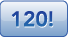Combinatorics - Calculate permutations, combinations, counting permutations, factorials and more.Probability - Binomial probability, hypergeometric probability, conditional probability, probability relation and Bayes Thereom.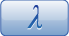Probability with time intervals - Calculate probability with time intervals with the geometric and negative binomial distribution.Expected Values - Use the expected values method to quickly find the expected value, variance and standard deviation.Confidence Intervals - Confidently and quickly calculate confidence intervals with a given confidence level.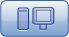Data Analysis - This computes tedious sums, squares of differences, and more to ultimately find the standard deviation and more.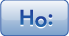Hypothesis Testing -- enter a few values and with the click of a mouse, know whether your null hypothesis is accepted.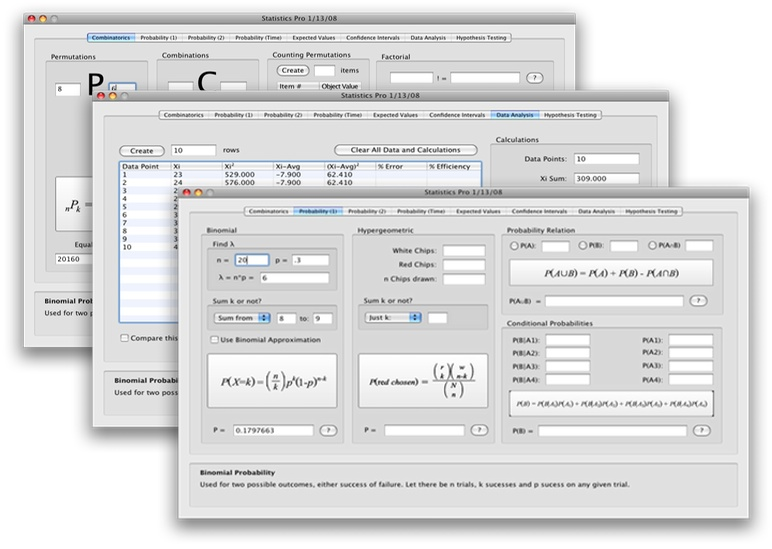A sampling of three tabs from Statistics Pro is shown above.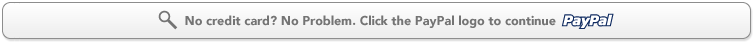a

 Macintosh Requirements PC Requirements Monitor resolution 1024x768 or higher REQUIRED Mac OS X Monitor resolution 1024x768 or higher REQUIRED Windows 2000-XP
 NOTE: If you have an older system than this, the software may still work. We encourage you to download the demo to try it.